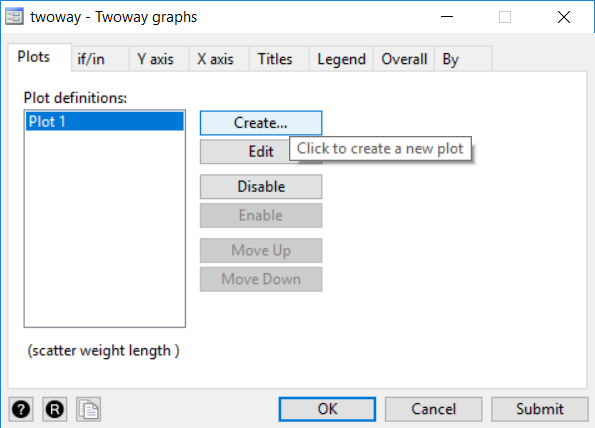Search

# Getting Started in Stata - Creating a Scatter Graph

Today we are going to show you how to create a scatter graph and add a line of best fit to it using both Stata menus and Stata commands. Graphics are an important tool for communicating data and results to others, as well as illustrating trends. The scatter graph is a common graph where you have an x (dependent) variable and a y (independent) variable and each observation is one point on the graph where the two variables intersect. You can use this graph to look at trends in your data and you can also add a trend line to your scatter graph.

As a new Stata user it is recommended that you start by using the Stata menus to perform your analysis and create graphs. Each analysis or graphic, such as a t-test or scatter graph, will show up in your Review pane (on the left side of the Stata screen) as the equivalent Stata command. This allows you to begin learning the general structure of commands and how to use them. Once you become more familiar with commands you will find it is faster and easier to perform your analyses using commands.

I am going to use the Stata Example dataset auto.dta. I load this dataset using the following command:

## Scatter Graph using Stata Menus (Part 1):

Graphics > Twoway graph (scatter, line, etc.)Click Create...Select “Basic plots” from the list of plot categories

Select/highlight “Scatter” under “Basic plots: (select types)”

Select “weight” as the Y variable and “length” as the X variable from the drop-down menus

Click “Accept”

Click “Submit”

Graphics > Twoway graph (scatter, line, etc.)Click Create… again to make a second plot (Plot 2)Select “Fit plots” from the list of plot categories

Select/highlight “Linear prediction” under “Fit plots: (select types)”

Select the same Y and X variables as you did for the first scatter plot

Click Accept

Click Submit

## Output:

For the first set of menu steps (Part 1) or the first Stata command above, you get the following graph:For the complete (parts 1 & 2) set of menu steps or the second Stata command above, you get the following graph:We expect to see a positive correlation between weight and length of cars, because as cars get longer we would expect them to also get heavier. We used the scatter graph to visually test this expectation and provide evidence that our assumption is correct. For this example there is a clear positive linear relationship between the x (length) and y (weight) variables, which is easily visible even without a line of best fit.

You can use scatter graphs to visually test the strength of relationships between variables, as we did here, but you can also use these graphs to look for patterns between variables when you aren’t aware of any relationships.